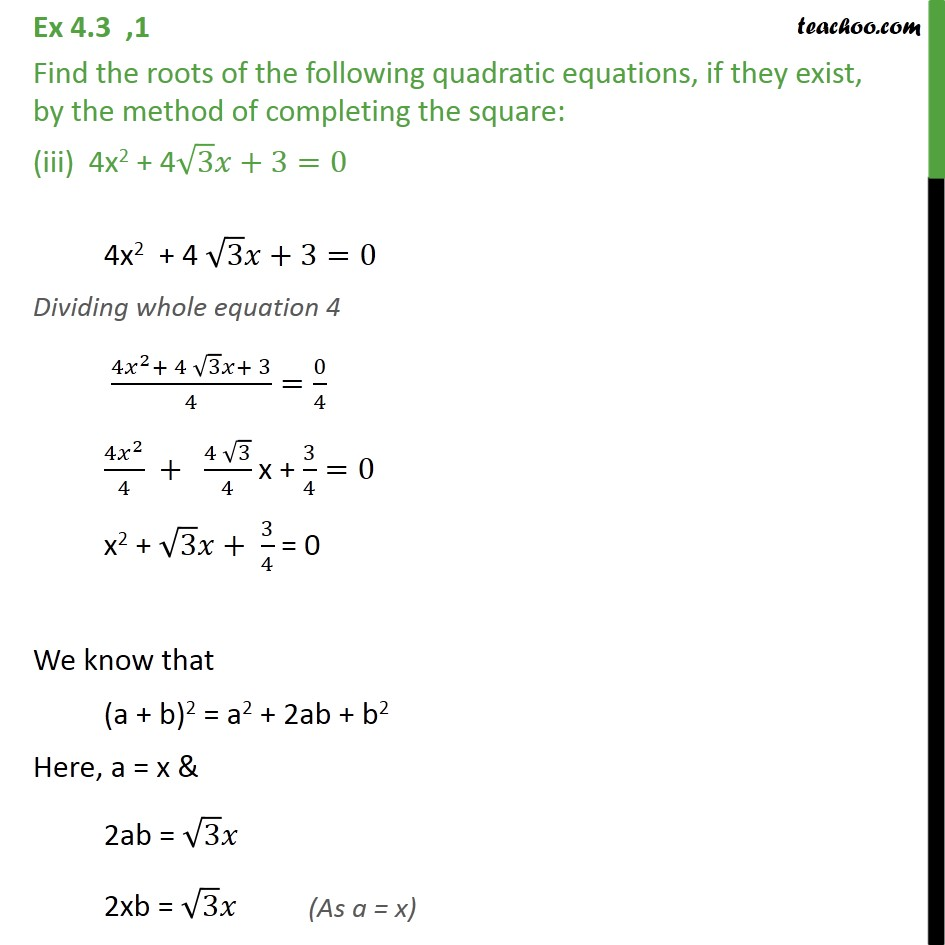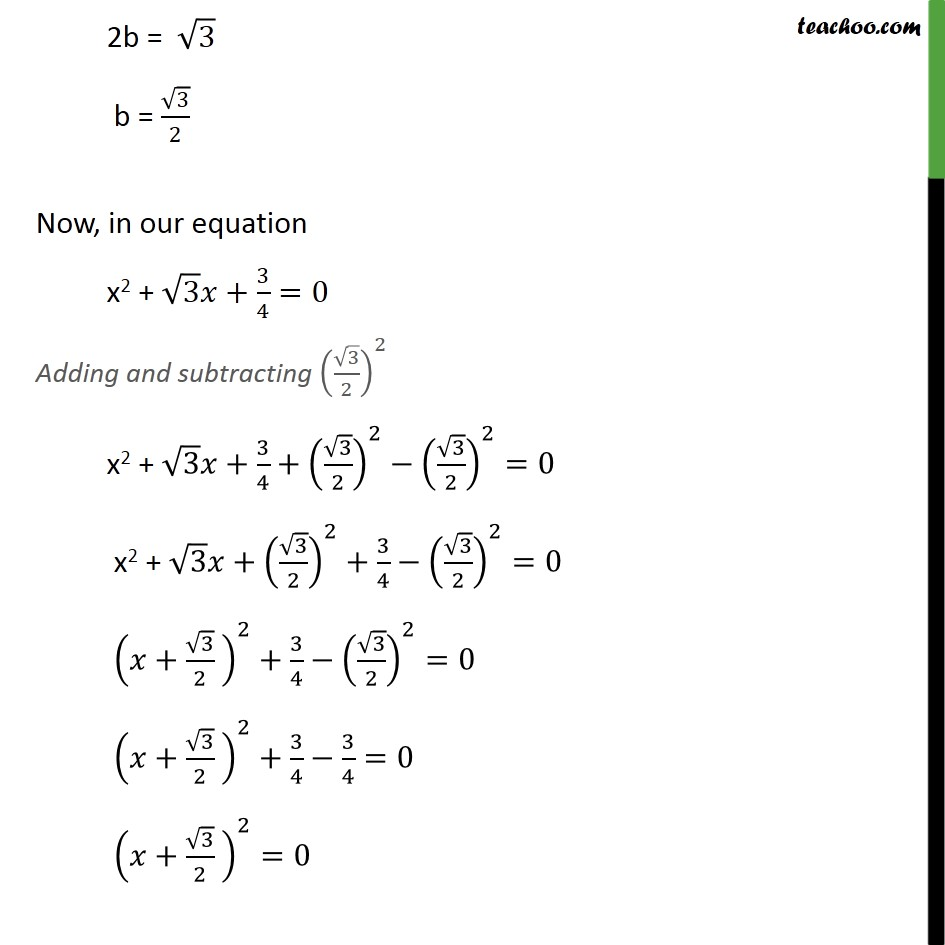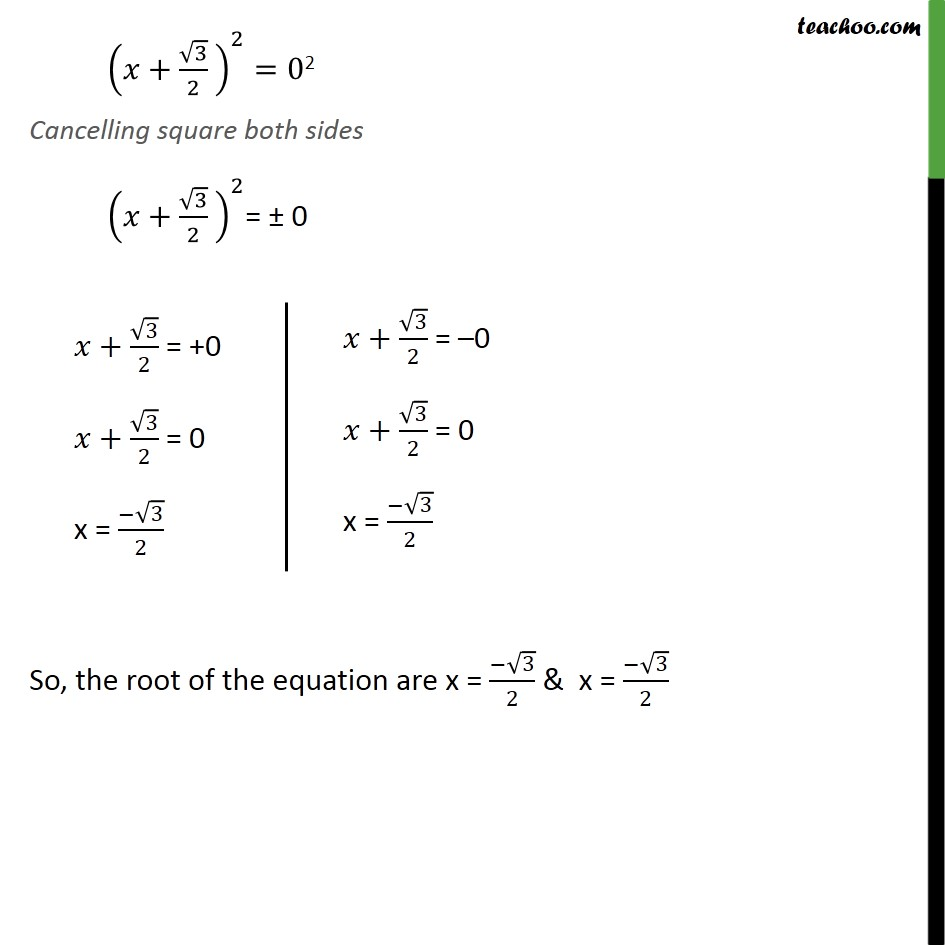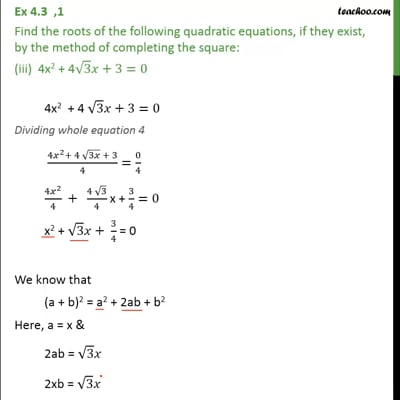Ex 4.3

Chapter 4 Class 10 Quadratic Equations (Term 2)
Serial order wiseThis video is only available for Teachoo black users

### Transcript

Ex 4.3 ,1 Find the roots of the following quadratic equations, if they exist, by the method of completing the square: (iii) 4x2 + 4√3 𝑥+3=0 4x2 + 4 √3 𝑥+3=0 Dividing whole equation 4 (4𝑥^2+ 4 √3 𝑥+ 3)/4=0/4 (4𝑥^2)/4 + (4 √3)/4 x + 3/4=0 x2 + √3 𝑥+ 3/4 = 0 We know that (a + b)2 = a2 + 2ab + b2 Here, a = x & 2ab = √3 𝑥 2xb = √3 𝑥 2b = √3 b = √3/2 Now, in our equation x2 + √3 𝑥+3/4=0 Adding and subtracting (√3/2)^2 x2 + √3 𝑥+3/4+(√3/2)^2−(√3/2)^2=0 x2 + √3 𝑥+(√3/2)^2+3/4−(√3/2)^2=0 (𝑥+√3/2 )^2+3/4−(√3/2)^2=0 (𝑥+√3/2 )^2+3/4−3/4=0 (𝑥+√3/2 )^2=0 (𝑥+√3/2 )^2=02 Cancelling square both sides (𝑥+√3/2 )^2= ± 0 So, the root of the equation are x = (−√3)/2 & x = (−√3)/2